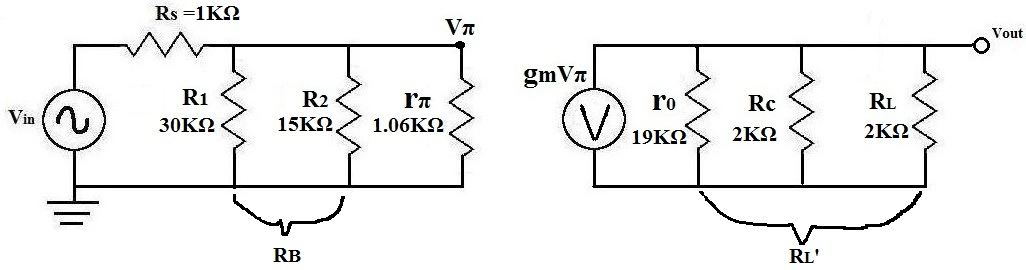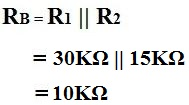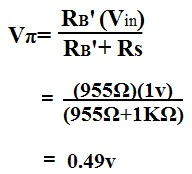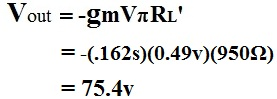﻿ Example of AC Transistor Circuit Analysis of the Mid Frequency Response

# Example- AC Transistor Circuit Analysis of the Mid Frequency ResponseAbove is a typical transistor circuit.

We will be solving for the mid frequency response of this transistor circuit.

AC Analysis
We will now perform AC analysis of the Mid Frequency Response of this transistor circuit to find its Midband Gain.

When doing AC analysis of the mid frequency response of this transistor circuit,
-All external capacitors (Cs, Ce, and Cc) are shorted
-Internal capacitors are open
So there is no capacitive effect (capacitors) in midband

Below is the equivalent AC Equivalent Circuit of the schematic above for finding its midband:Analysis
Right away, we can begin doing analysis on the circuit to narrow it down and begin calculating values:

Solve R1||R2 (which is RB)

The first thing to do is solve for RB:Solve for RB||rπ
Next, after you get the value for RB, solve for RB', which is RB||rπ. The value of rπ is obtained from doing DC analysis of the transistor circuit. To learn how to calculate rπ, read How to Calculate rπ of a Transistor.Solve for Output Resistance RL'
Next, we solve for the output resistance of the transistor circuit, RL', which equal to r0 || Rc || RL.Solve for Vπ
Next, we solve for Vπ, which is the voltage that drops across rπ. This is important for other calculations in the AC analysis.Once we have these calculations, we can reduce the above circuit to the one below:Now we can solve for Vout and then the gain of the transistor, Am. The value of Gm is obtained from doing DC analysis of the transistor circuit. To learn how to calculate gm, read How to Calculate Gm of a Transistor.We now solve for the midband gain of the transistor circuit, Am: